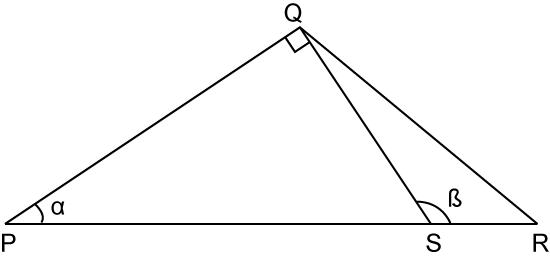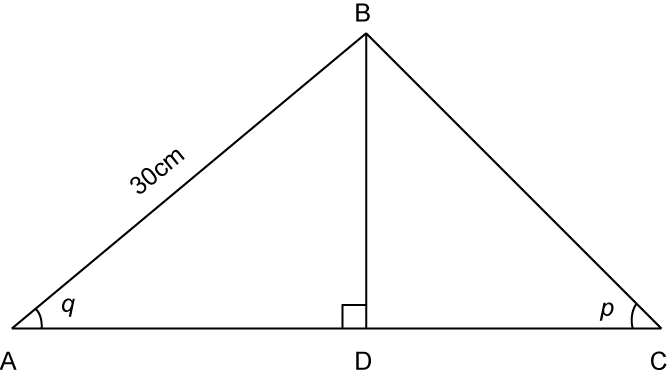# 6.3.2 Ratio and Graphs of Trigonometric Functions, SPM Paper (Short Questions)

Question 4:In the diagram above, WZY  is a straight line.  $\angle XYZ={90}^{o},\text{}\angle XWZ={30}^{o}$ and WZ = XZ = 30 cm. Find the length of XY.

Solution:
$\begin{array}{l}\angle WXZ=\angle XWZ={30}^{o}\\ \therefore \angle XZY={30}^{o}+{30}^{o}={60}^{o}\\ \\ \mathrm{sin}\angle XZY=\frac{XY}{XZ}\\ \mathrm{sin}{60}^{o}=\frac{XY}{30}\\ XY=\mathrm{sin}{60}^{o}×30\\ XY=25.98cm\end{array}$

Question 5:In the diagram above, PQS is a right angle triangle. Given that SR = 6cm, PQ = 12 cm and 5SR = 2PS. Find the value of cos α and tan β.

Solution:

Question 6:In the diagram above, ADC is a straight line, if  $\mathrm{sin}q=\frac{3}{5}\text{and}\mathrm{tan}p=\frac{1}{2}$ . Find the distance of AC.

Solution:
$\begin{array}{l}\text{Given}\mathrm{sin}q=\frac{BD}{AB}=\frac{3}{5}\\ \frac{BD}{30}=\frac{3}{5}\\ BD=\frac{3}{5}×30\\ BD=18\text{}cm\\ \\ \text{In}△\text{}ABD,\text{using Pythagoras’ Theorem,}\\ AD=\sqrt{A{B}^{2}-B{D}^{2}}\\ AD=\sqrt{{30}^{2}-{18}^{2}}=24\text{}cm\\ \\ \text{Given tan}p=\frac{BD}{DC}=\frac{1}{2}\\ \frac{18}{DC}=\frac{1}{2}\\ DC=36\text{}cm\\ \\ \text{Hence, distance of}AC=24+36=60\text{}cm.\end{array}$

### 4 thoughts on “6.3.2 Ratio and Graphs of Trigonometric Functions, SPM Paper (Short Questions)”

1.Hi, for question 5 the answer for cos alpha is 4/5 not 3/5. Please correct the simplification.

•Dear Gurdit Singh,
thanks for pointing out our mistake, correction had been made accordingly.

2.question 5 cos alpha should be 4/5 and not 3/5 as 12/15(which is correct) equals to 4/5

•Dear donald,
thanks for pointing out our mistake, correction had been made accordingly.